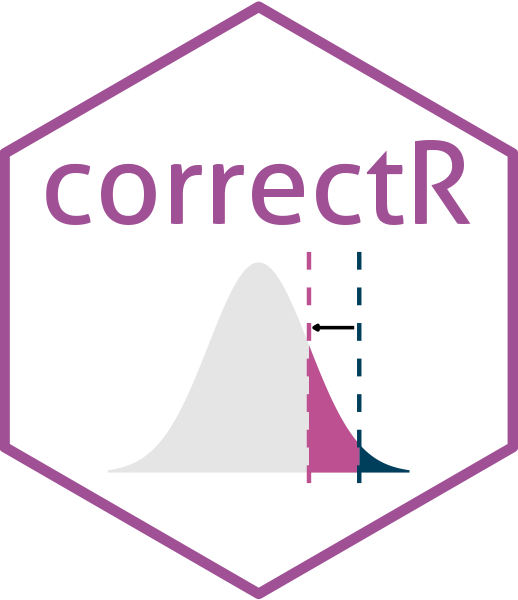# correctRCorrected test statistics for comparing machine learning models on correlated samples

## Installation

You can install correctR from GitHub:

devtools::install_github("hendersontrent/theft")

## General purpose

Often in machine learning, we want to compare the performance of different models. However, the methods used to obtain these performance metrics (e.g., classification accuracy) violate the assumptions of traditional statistical tests such as a $$t$$-test. Examples of these methods include data resampling and $$k$$-fold cross-validation. The purpose of these methods is to either aid generalisability of findings (i.e., through quantification of error as they produce multiple values for each model instead of just one) or to optimise model hyperparameters. This makes them invaluable, but unusable with comparative approaches such as a $$t$$-test, as Dietterich (1998) found that the standard $$t$$-test underestimates the variance, therefore driving a high Type I error. correctR is a lightweight package that implements a small number of corrected test statistics for cases when samples are not independent (and therefore are correlated), such as in the case of resampling and $$k$$-fold cross-validation. These corrections were all originally proposed by Nadeau and Bengio (2003). Currently, only cases where two models are to be compared are supported.